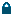Log In
 EN CHOOSE LANGUAGE ENGLISH
 MONEY MANAGEMENT What is the minimum required deposit? What is a pip and what is its value?
 HELP CATEGORY: SIGNALS > MONEY MANAGEMENT > What is a pip and what is its value?

# What is a pip and what is its value?

A pip is the smallest change of a currency pair rate. As an example we will use the EUR/USD currency pair rate with 4 and 5 digits with 1.2353 and 1.23531 respectively. If your broker provides a four digit price, a one pip change will be 0.0001 whilst for a five digit rate it will be 0.00001.

To calculate a value of a pip, we have to understand what a pip is in terms of real money. Any currency rate is a ratio of one currency value to another. For example, 1.6357 GBP/USD means 1 Great Britain Pound (GBP) equals 1.6357 United States Dollars (USD). GBP is the first currency, and USD is the second currency within the GBP/USD currency pair.

Trading forex is actually buying and selling a certain amount of a currency. You operate with lots (standard, mini and micro lots). The standard lot is 100,000 units of the first currency in a currency pair. Trading through brokers allows you to open 100,000 units (1.0 standard lot) having only \$1,000 by means of leverage (1:100 in this case).

The money value of a pip depends on how much you have opened (or how many lots / units you are trading with). Let us assume, we buy (open a position) GBP/USD at 1.6437 with 1.0 standard lot and sell it back (close the position) at 1.6489. The profit in pips will be = 1.6489 - 1.6437 = 0.0052 or 52 pips. In terms of money, the profit will be 52 * \$10 = \$520. It is derived from the simple calculation: when we buy 1 standard lot, we spend 164,370 USD to buy 100,000 GBP. At the time we sell (close the position), the rate is 1.6489 which means the 100,000 GBP we have, will be converted back to USD and we will receive \$164,890. As we paid initially \$164,370, the difference is our profit = \$520. If we had opened only 0.1 lot the profit would have been only \$52.

 Page statistics. Views: 1188 Last updated: 22 June 2016